# 0x01 赛题说明# 0x02 曲折

• 直接右击txt打开，查找关键词 flag
• 查看该音频文件的波形图、频谱图，是否存在相关信息可以转化为摩斯电码
• 查看mp3 中是否含有隐藏文件，提取文件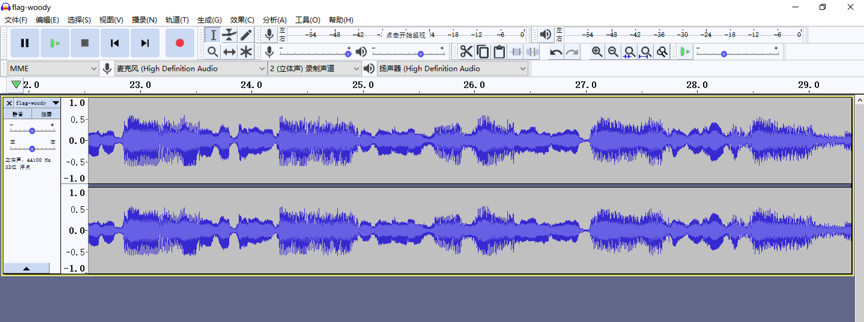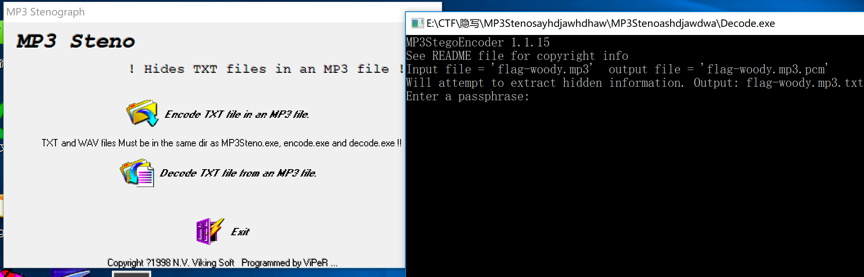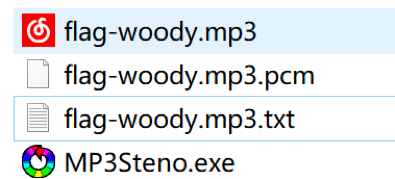Binwalk 发现：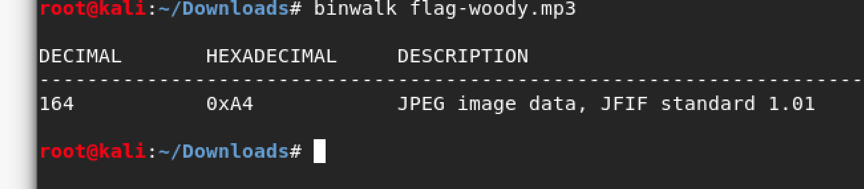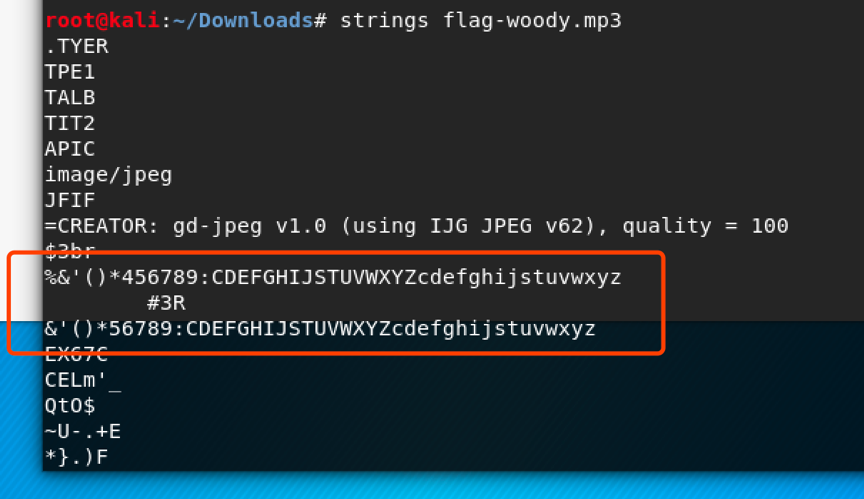# 0x03 题解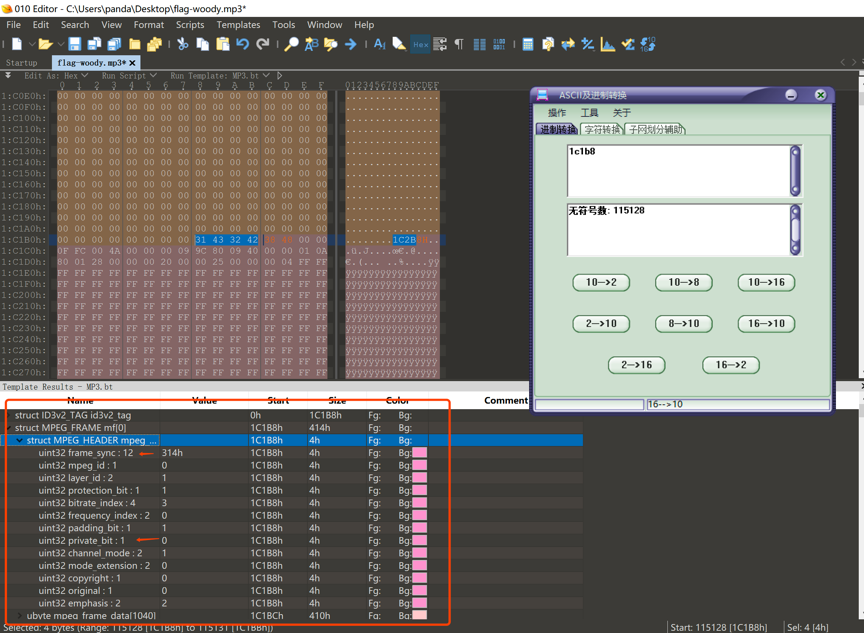``````uint32 frame_sync : 12

uint32 mpeg_id : 1

uint32 layer_id : 2

uint32 protection_bit : 1

uint32 bitrate_index : 4

uint32 frequency_index : 2

uint32 private_bit : 1

uint32 channel_mode : 2

uint32 mode_extension : 2

uint32 original : 1

uint32 emphasis : 2``````

12+1+2+1+4+2+1+1+2+2+1+1+2=32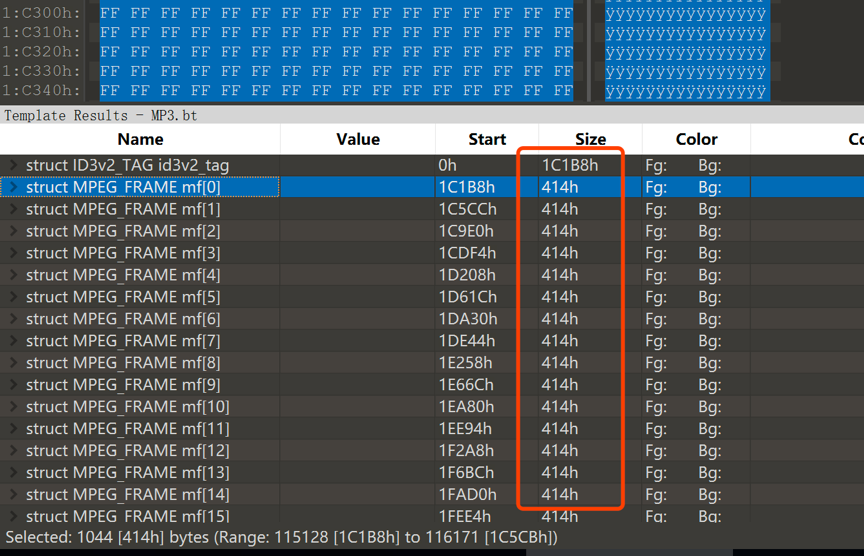``````# coding:utf-8
import re
import binascii

n = 115130
result = ''
fina = ''
file = open('flag-woody.mp3','rb')
while n < 2222222 :
file.seek(n,0)
n += 1044
textArr = re.findall('.{'+str(8)+'}', result)
textArr.append(result[(len(textArr)*8):])
for i in textArr:
fina = fina + hex(int(i,2))[2:].strip('\n')
fina = fina.decode('hex')
print fina``````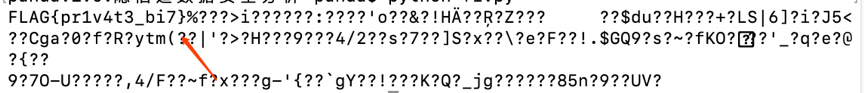「一键投喂 软糖/蛋糕/布丁/牛奶/冰阔乐！」

(๑＞ڡ＜)☆谢谢老板~